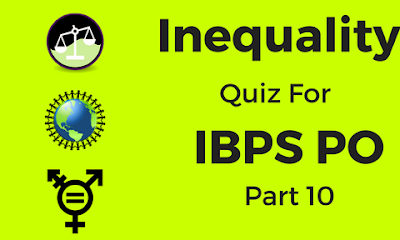Gurpurab Offer - Use Code GURPURAB2023

# Inequality Quiz For IBPS PO - Part 10Direction(Q1-5): In the following questions, the symbols %, #, @, & and \$ are used with the following meaning as illustrated below:
‘P % Q’ means ‘P is not greater than Q’.
‘P \$ Q’ means ‘P is neither greater than nor equal to Q’.
‘P & Q’ means ‘P is neither greater than nor smaller than Q’
‘P < Q’ means ‘P is neither smaller than nor equal to Q’
‘P > Q’ means ‘P is not smaller than Q’

### Question 1.

In Which of the following expressions does the expression 'J \$ K' to be definitely hold true?
A. J & P < L > K % M
B. J \$ P > L & M % K
C. J % P & L \$ K % M
D. J > P & L % M \$ K
E. None of these
Ans. C.
Solution:
J<K
J=P>L≥K≤M
J<P≥L=M≤K
J≤P=L<K≤M
J≥P=L≤M<K

### Question 2.

In Which of the following expressions does the expression 'A % B' to be definitely hold true?
A. C \$ A \$ D & B < E
B. A < D \$ B % C > E
C. D & B % C & A < E
D. E \$ A % C & D % B
E. None of these
Ans. D.
Solution:
A≤B
C<A<D=B>E
A>D<B≤C≥E
D=B≤C=A>E
E<A≤C=D≤B

### Question 3.

Which of the following symbols should replace the question mark (?) respectively in order to complete the given expression in such a manner that both P%S, as well as Q\$M, definitely holds true?
P % Q & R ? S \$ T, M ? R > U \$ V
A. \$, &
B. \$, \$
C. %, >
D. %, <
E. None of these
Ans. D.
Solution:
P≤S, Q<M
P≤Q=R<S<T, M=R≥U<V
P≤Q=R<S<T, M<R≥U<V
P≤Q=R≤S<T, M≥R≥U<V
P≤Q=R≤S<T, M>R≥U<V

### Question 4.

Which of the following expressions is false if the expression 1%3<4&5%6\$8<2\$7 is definitely true?
A. 4\$8
B. 4%6
C. 3<5
D. 6\$3
E. None of these
Ans. D.
Solution:
1≤3>4=5≤6<8>2<7
4<8
4≤6
3>5
6<3

### Question 5.

L < K \$ M % N & K & O \$ J
Conclusions: L \$ N, O % M
A. Only I is true
B. Only II is true
C. Either I or II is true
D. Both I and II are true
E. Neither I nor II is true.
Ans. E.
Solution:
L>K<M≤N=K=O<J
L<N, O≤M

### Question 6.

In which of the following expressions does the expression 'H<I' to definitely hold true?
A. G>H>O≤M, O=S≤P<I
B. G>M<I≥N<O, P≤M>Q≤H
C. G<S≥M<N=I, P≤O=M≥T>H
D. G<I<O>M=P, T≤N>H<M
E. None of these
Ans. C.
Solution:
From C we got H<I.
I=N>M≥T>H

### Question 7.

Which of the following symbols should replace the question mark (?) respectively in order to complete the given expression in such a manner that both A<F, as well as G≥D, definitely holds true?
F?G≥C=H≥I, A≤B<C?D>E
A. =, <
B. >, ≤
C. ≤, >
D. >, =
E. None of these
Ans. D.
Solution:
A. A<F (F=G≥C>B≥A), G≥D (G≥C<D>E)
B. A<F (F>G≥C>B≥A), G≥D (G≥C≤D>E)
C. A<F (F≤G≥C>B≥A), G≥D (G≥C>D>E)
D. A<F (F>G≥C>B≥A), G≥D (G≥C=D>E)

### Question 8.

Which of the following expressions is false if the expression J<K≥L>M=N<O, P<Q>L≥R>S is definitely true?
A. J<S
B. P≥K
C. S>M
D. R=N
E. K≥R
Ans. E.
Solution:
J<S (J<K≥L≥R>S)
P≥K (P<Q>L≤K)
S>M (M<L≥R>S)
R=N (R≤L>M=N)
K≥R (K≥L≥R)

### Question 9.

Which of the following symbols should replace the blank space respectively in order to complete the given expression in such a manner that both G≥E, as well as J>H, definitely holds true?
E_F_G_H_I_J
A. =, ≥, >, =, ≤
B. >, ≥, ≤, <, ≤
C. <, =, >, >, =
D. <, ≤, <, <, =
E. ≤, =, <, <, ≤
Ans. E.
Solution:
E=F≥G>H=I≤J
E>F≥G≤H<I≤J
E<F =G>H>I=J
E<F≤G<H<I=J
E≤F=G<H<I≤J

### Question 10.

Which of the following expressions is false if the expression P≤Q=R≥S>T, M>N=R<O≤L is definitely false?
A. Q<O
B. M≥S
C. L>T
D. N=Q
E. None of these
Ans. B.
Solution:
Q<O (Q=R<O)
M≥S (M>N=R≥S)
L>T (T<S≤R<O≤L)
N=Q (Q=R=N)